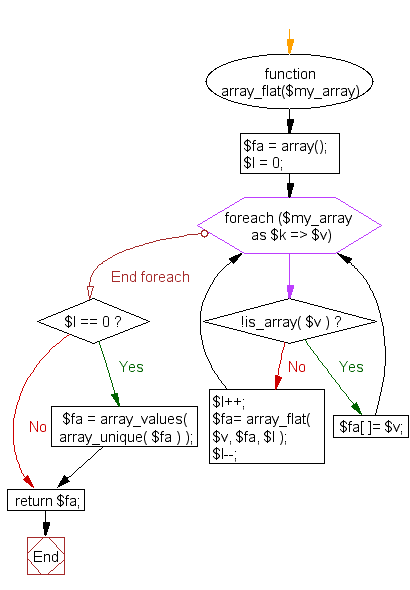﻿ PHP Array Exercise: Find unique values from multidimensional array and flatten them in zero depth - w3resource# PHP Array Exercises : Find unique values from multidimensional array and flatten them in zero depth

## PHP Array: Exercise-42 with Solution

Write a PHP function to find unique values from multidimensional arrays and flatten them in 0 depth.

Sample Solution:

PHP Code:

``````<?php
function array_flat(\$my_array)
{
\$fa = array();
\$l = 0;
foreach(\$my_array as \$k => \$v )
{
if( !is_array( \$v ) )
{
\$fa[ ]= \$v;

continue;
}
\$l++;
\$fa= array_flat( \$v, \$fa, \$l );
\$l--;
}

if( \$l == 0 ) \$fa = array_values( array_unique( \$fa ) );
return \$fa;
}
\$tmp = array( 'a' => array( -1,-2, 0, 2, 3 ), 'b' => array( 'c' => array( -1, 0, 2, 0, 3 ) ) );
print_r(array_flat(\$tmp));
?>
```
```

Sample Output:

```Array
(
 => -1
 => 0
 => 2
 => 3
)
```

Flowchart:PHP Code Editor:

What is the difficulty level of this exercise?

﻿

## PHP: Tips of the Day

PHP: Extract numbers from a string

```\$str = 'In My Cart : 11 12 items';
preg_match_all('!\d+!', \$str, \$matches);
print_r(\$matches);
```